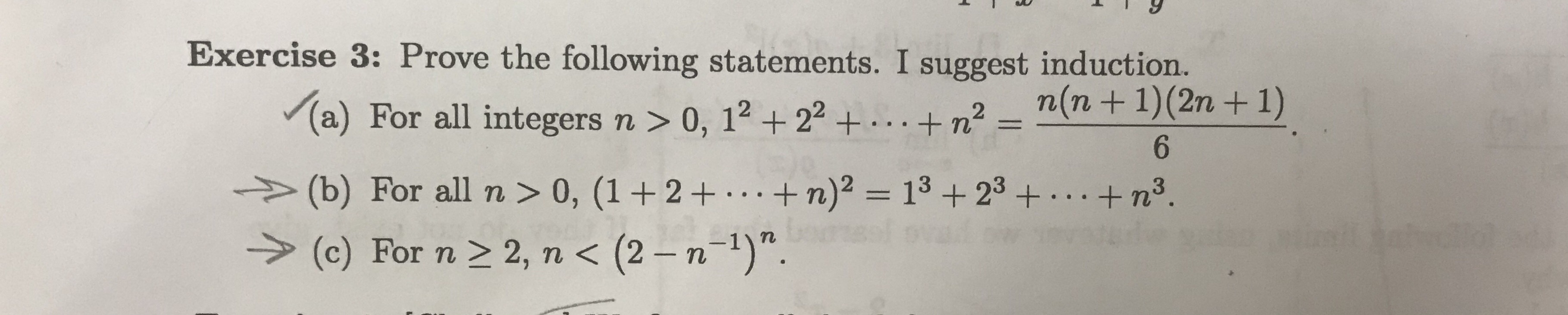# Exercise 3: Prove the following statements. I suggest induction.-n(n+1)(2n +1)(a) For all integers n > 0, 12 2 ++n2(b) For all n > 0, (1 + 2+ . ..+ n)2 = 13 + 23 +.+n3.2, n < (2 - n-1)".(c) For n

Question
1 views

Prove b and c by inductionhelp_outlineImage TranscriptioncloseExercise 3: Prove the following statements. I suggest induction. -n(n+1)(2n +1) (a) For all integers n > 0, 12 2 ++n2 (b) For all n > 0, (1 + 2+ . ..+ n)2 = 13 + 23 +.+ n3. 2, n < (2 - n-1)". (c) For n fullscreen
check_circle

Step 1

“Since you have asked multiple question, we will solve the first question for you.Please post the another question separately."

Step 2

We shall prove the result (1+2+.....n)^2=1^3+2^3+.......n^3 ,where n is integer>0 ,by using principle of mathematical induction.

Step 3

Step 1) We shall prove the resu...

### Want to see the full answer?

See Solution

#### Want to see this answer and more?

Solutions are written by subject experts who are available 24/7. Questions are typically answered within 1 hour.*

See Solution
*Response times may vary by subject and question.
Tagged in

### Other